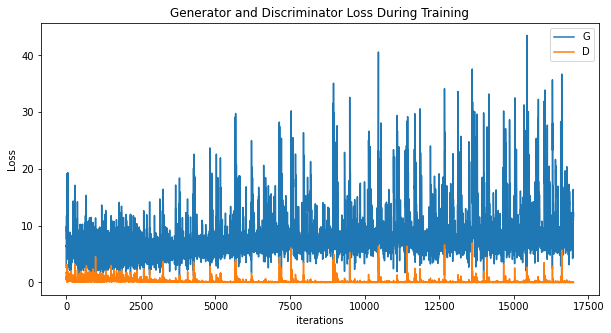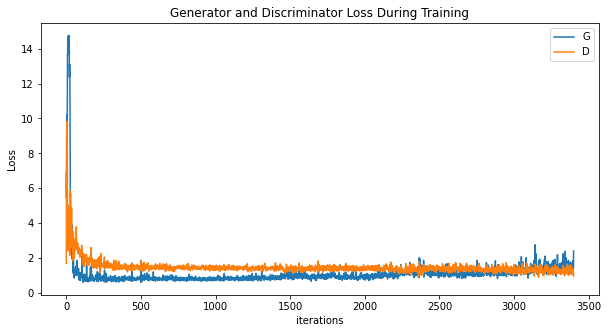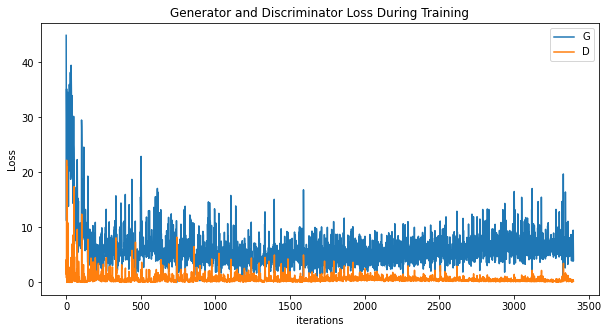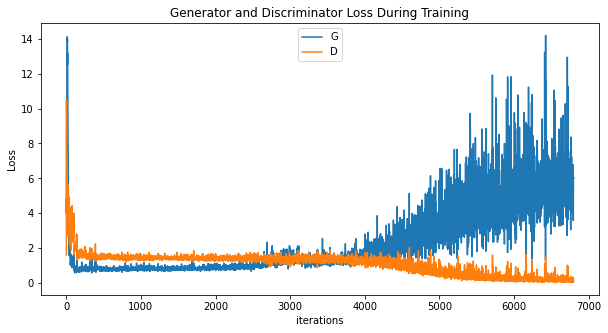# What can be inferred from following GAN loss plots?

I am trying out different hyperparameter tunings like changing the number of epochs and using Two Time-Scale update rule.(different Learning rates for Discriminator (D_lr) and Generator (G_lr)).

Was going through some medium articles and I did few trials and have generated some plots for the same

Plot 1 - Ran for 500 Epochs (same learning rate of 0.0002 for both G and D)Plot 2 - Ran for 100 Epochs with D_lr = 0.0002 and G_lr = 0.002Plot 3 - Ran for 100 Epochs with D_lr = 0.002 and G_lr = 0.0002Plot 4 - Ran for 200 Epochs with D_lr = 0.0002 and G_lr = 0.002I am a little confused with regard to what these plots convey.

Plot 1 and plot 3 seem to indicate similar results although TTUR was not applied to plot 1.

The plot 2 and plot 4 seem to indicate similar initial trends but in plot 4 around 3500 iterations, G and D loss seem to branch out.

What can be the reason for this behavior?

PS
I am new to the world of GAN
The size of dataset was around 1100 images - pretty low for a GAN training